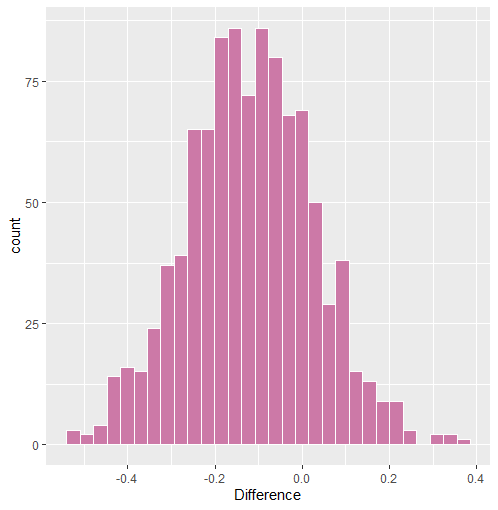Introduction

This is a follow-up to the vignette “Three Ways to Test the Same Hypothesis”. A new feature, pcor_sum, was added to BGGM that allows for testing partial correlation sums. This differs from the Bayes factor approach (“Approach #3”), in that only the posterior distribution is used to determine whether there is a difference in the sums.

R package

# need the developmental version
if (!requireNamespace("remotes")) {
install.packages("remotes")
}

# install from github
remotes::install_github("donaldRwilliams/BGGM")
library(BGGM)

One Group

This first example looks at one group, where a sum is tested within the same ptsd network. I focus on the relations between the re-experiencing (B) and avoidance (C) communities. In particular, the sum of relations between the “Intrusion” (5 nodes) community and the “Avoidance” (two nodes) community is tested.

Sum to String

For the avoidance symptom “avoidance of thoughts” C1, this can be written in R code with

# ptsd
Y <- ptsd

# paste together sums
paste0(colnames(Y)[1:5],  "--C1", collapse = " + ")

#> "B1--C1 + B2--C1 + B3--C1 + B4--C1 + B5--C1"

whereas, for the avoidance symptom “avoidance of reminders” (C2), this is written as

paste0(colnames(Y)[1:5],  "--C2", collapse = " + ")

#> "B1--C2 + B2--C2 + B3--C2 + B4--C2 + B5--C2"

Note that typically this would have to be written out. paste0 was used in this case to avoid typing out all of the relations.

Fit Model

Here an ordinal GGM is fitted

fit <- estimate(Y+1, type = "ordinal", iter = 1000)

where the +1 changes the first category from 0 to 1 (required).

Test Sums

The next step is to use the pcor_sum function. First, I combine the sums into one string separated with ;.

# sum 1
sum1 <- paste0(colnames(Y)[1:5],  "--C1", collapse = " + ")

# sum 2
sum2 <- paste0(colnames(Y)[1:5],  "--C2", collapse = " + ")

# paste together
sums <- paste(sum1, sum2, sep = ";")

# print
sums
#> "B1--C1 + B2--C1 + B3--C1 + B4--C1 + B5--C1;B1--C2 + B2--C2 + B3--C2 + B4--C2 + B5--C2"

Next pcor_sum is used

test_sum <- pcor_sum(fit, relations = sums)

# print
test_sum

# BGGM: Bayesian Gaussian Graphical Models
# ---
# Network Stats: Posterior Sum
# Posterior Samples: 1000
# ---
# Estimates
#
# Sum:
#                                    Post.mean Post.sd Cred.lb Cred.ub
# B1--C1+B2--C1+B3--C1+B4--C1+B5--C1     0.215   0.096   0.034   0.404
# B1--C2+B2--C2+B3--C2+B4--C2+B5--C2     0.334   0.097   0.145   0.514
# ---
#
# Difference:
# B1--C1+B2--C1+B3--C1+B4--C1+B5--C1 - B1--C2+B2--C2+B3--C2+B4--C2+B5--C2
#
#  Post.mean Post.sd Cred.lb Cred.ub Prob.greater Prob.less
#     -0.119   0.145  -0.409   0.173        0.205     0.795
# ---

Prob.greater is the posterior probability that the first sum is larger than the second sum.

Plot Results

The object test_sum can then be plotted. Note this returns three plots, but only the difference is shown here

plot(test_sum)\$diffThe histogram is not very smooth in this case because iter = 1000, but this of course can be changed.

Two Groups

This next example is for two groups. The data are called bfi and they are in the BGGM package. I compare a sum of two relations for questions measuring agreeableness in males and females. The relations tested are as follows

Sum to String

sums <- c("A3--A4 + A4--A5")

where A1 is “know how to comfort others,” A4 is “love children,” and A5 is “make people feel at ease.”

Fit Models

The next step is to fit the models

# data
Y <- bfi

# males
Y_males <- subset(Y, gender == 1, select = -c(education, gender))[,1:5]

# females
Y_females <- subset(Y, gender == 2, select = -c(education, gender))[,1:5]

fit_female <- estimate(Y_females, seed = 2)

# fit males
fit_male <- estimate(Y_males, seed = 1)

Test Sums

Then test the sum

test_sum <- pcor_sum(fit_female, fit_male, relations = sums)

# print
test_sum

#> BGGM: Bayesian Gaussian Graphical Models
#> ---
#> Network Stats: Posterior Sum
#> Posterior Samples: 5000
#> ---
#> Estimates
#>
#> Sum:
#>                   Post.mean Post.sd Cred.lb Cred.ub
#> g1: A3--A4+A4--A5     0.292   0.026   0.241   0.342
#> g2: A3--A4+A4--A5     0.305   0.036   0.234   0.375
#> ---
#>
#> Difference:
#> g1: A3--A4+A4--A5 - g2: A3--A4+A4--A5
#>
#>  Post.mean Post.sd Cred.lb Cred.ub Prob.greater Prob.less
#>     -0.014   0.045    -0.1   0.074        0.386     0.614
#> ---

Sanity Check

For a kind of sanity check, here is the sum for the male group obtained from the point estimates.

pcor_mat(fit_male)["A3", "A4"] + pcor_mat(fit_male)["A4", "A5"]

#>  0.305

This matches the output.